# Data Visualization in R Interview Questions and Answers BI and Visualization

• 4.6 Rating
• 50 Question(s)

## Intermediate

This is one of the most frequently asked data visualization in R interview questions for freshers in recent times.

Following “libraries/packages in R” are typically used for data visualization purposes and also quite useful with their usage and features.

ggplot2, Lattice, Leaflet, Highcharter, RColorBrewer, plotly, sunburstR, RGL, dygraphs

Out of the above “ggplot2” is extremely popular and some of the sources indicate that this is one of the highest downloaded packages by users for the purpose of data visualization/graphics using R packages.

• ggplot2 – is an implementation of the grammar of graphics and can be used for custom plots using R. While it is simple to create standard plots or charts in R, ggplot2 is used to build “custom” plots in a simple manner which are difficult to create without the usage of this library. We can use this library to build plots in a systematic fashion – i.e. create our plot with axes, then go on to add points, then go on to add a line, then add some statistical inference metric such as confidence interval, then highlight a regression curve with some mathematical equation in the background and so on.
• RColorBrewer – is a library on colour brewer palettes. It provides colour schemes for maps. It can be used to manipulate colours in plots/charts, graphs, maps etc. This is designed by Cynthia Brewer. It can be used along with “plotly” package as well.
• Leaflet – is basically used for maps. We can create interactive maps leveraging this. The interface for a leaflet in R is using the “htmlwidgets” framework. Hence it can be managed in markdown documents easily and also in shiny UI applications.

It is simple and easy to create multiple plots onto a single page using R. The following syntax can be used to capture a 2 X 2 plot in a single page.

par(mfrow=c(2,2))

For example, if we want to display histogram charts for IRIS dataset for various sepal and petal width and lengths, then each of the below commands will display one of the histogram charts on one page using R.

hist(iris$Sepal.Length) hist(iris$Sepal.Width) hist(iris$Petal.Length) hist(iris$Petal.Width)

Now if we use the command par(mfrow=c(2,2)) and then execute about code for plotting histogram, then four charts are displayed in a 2 X 2 format (2 rows with 2 columns). A sample representation of the result is shown in the below diagram.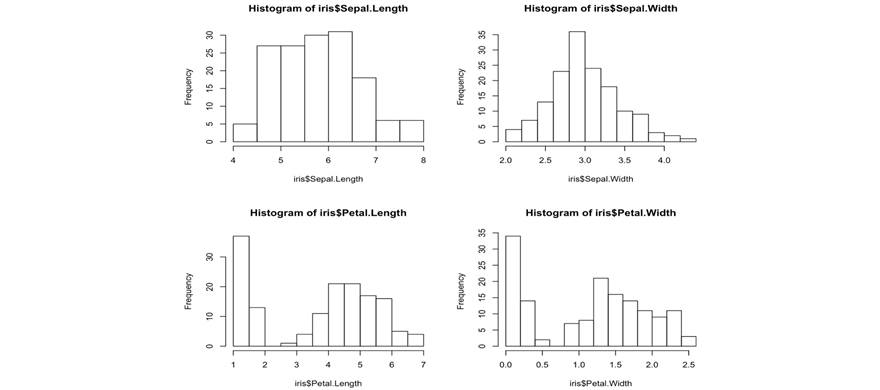Similarly, 3X3 representation can be displayed using something like this - par(mfrow=c(3,3)) and so on.

Lattice is a powerful and high-level data visualization system inspired by trellis graphics for R. This is used with an emphasis to deal with multivariate data. This is contributed by a person named Deepayan Sarkar.

We can take the mtcars dataset (car dataset with parameters such as mileage, weight, number of gears, number of cylinders etc.) for demonstrating some sample visualizations leveraging this package.

Density plot and scatter plot matrix can be drawn by leveraging this library.

# kernel density plot densityplot(~mpg,

main="Density Plot", xlab="Miles per Gallon")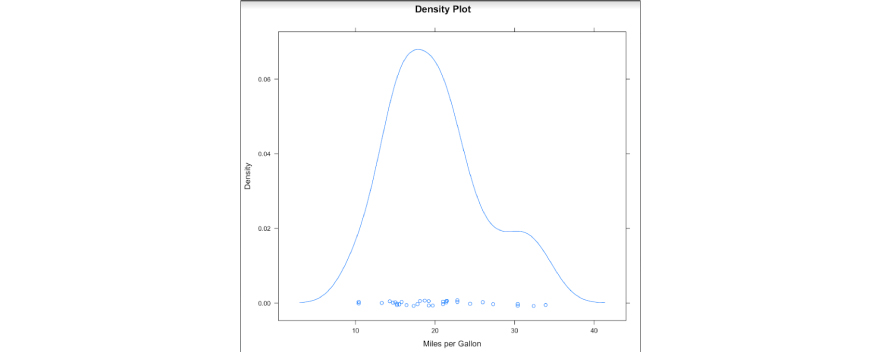# scatterplot matrix splom(mtcars[c(1,3,4,5,6)],main="MTCARS Data")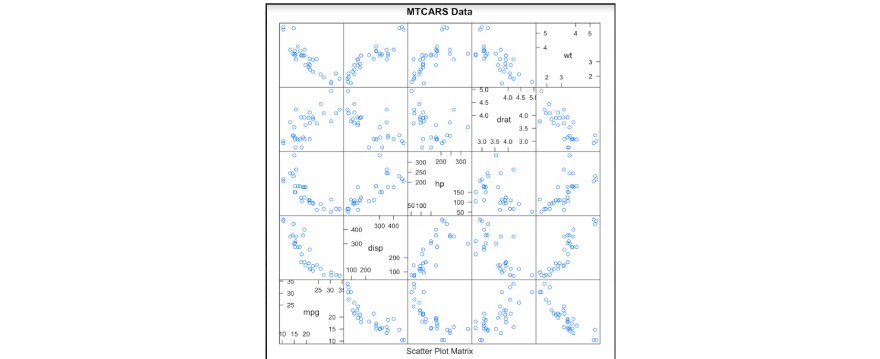Ggplot2 package
Lattice package
It uses counts, not percentages by
default.

It plots the facets starting from top-left.
It plots the facets starting from the bottom-left.
Ggplot2 orders facets in the opposite
direction compared to that in lattice

Sorting each facet separately is not possible in ggplot2

A scatter plot is a chart used to plot a correlation between two or more variables at the same time. We can consider the example of IRIS dataset in R using ggplot2 library.

# Example of ScatterPlot library(ggplot2)

ggplot(iris,aes(y=Sepal.Length,x=Petal.Length))+geom_point() Sample output: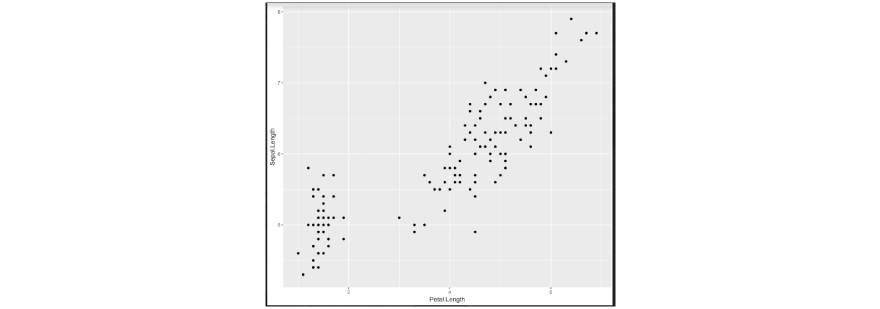This shows a comparison between Sepal. Length and Petal.Length in the IRIS dataset leveraging R ggplot2 library.

We use a histogram to plot the distribution of a continuous variable, while we can use a bar chart to plot the distribution of a categorical variable.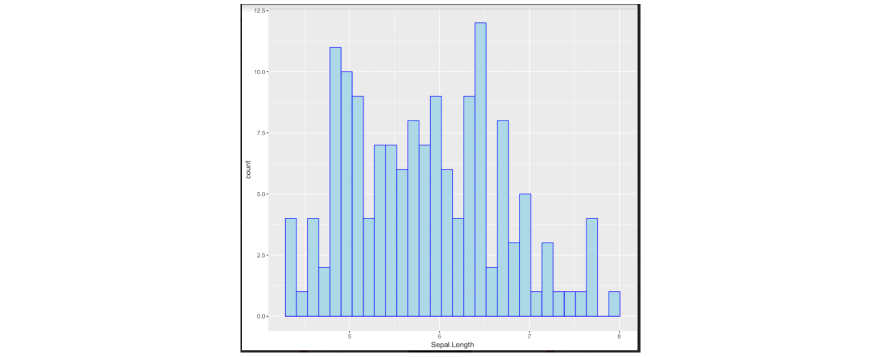Let us take the example of IRIS dataset in R.

We will plot a histogram of IRIS dataset with leveraging “ggplot2” package in R. “Sepal.Length” is a continuous variable which is plotted below onto the x-axis.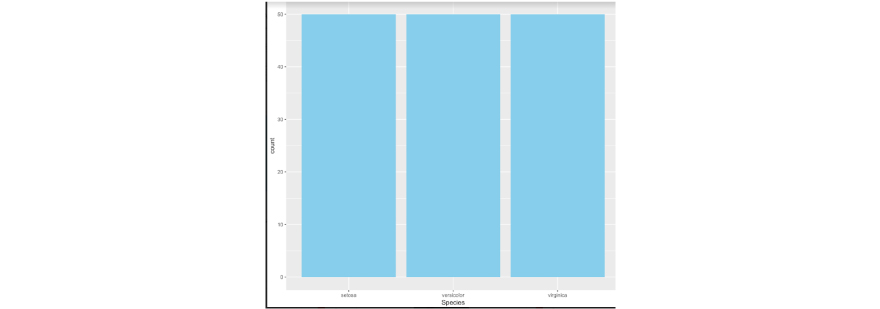Code:

ggplot(data = iris,aes(x=Sepal.Length))+geom_histogram(fill="lightblue",col="blue")

We will plot a bar chart of IRIS dataset with leveraging “ggplot2” package in R. “Species” is a categorical variable which is plotted below onto the x-axis.

Code:

ggplot(data = iris,aes(x=Species))+geom_bar(fill="skyblue")

A time-series is a plot where all the measurements are plotted sequentially. Time here is represented along the x-axis while the variable of interest is a plot on the y-axis. For many data, among which environmental observations, taking a look at their temporal pattern may be extremely useful for gaining insight into their behaviour.

In many cases, the variable time is underestimated. However, time-series are extremely useful to determine the temporal pattern of a variable.

We take an example of sample dataset called “nottem” in R which captures average monthly temperatures at Nottingham, between 1920 to 1939.

The chart shows x1 (which is the average temperature of the city) over a period of time for around 19-20 years.

Response:

We could easily save our plots as images directly from R using an editor such as RStudio. This way of saving, however, does not provide much flexibility. If we want to customize our images, we need to have an approach as to how to export plots from the R code itself.

We can use “ggsave” function to accomplish this.

We can save the plots in different formats such as jpeg, tiff, pdf, svg etc. We can also use various parameters to change the size of the image prior to exporting it or saving it in a path or location.

# Saving as jpeg format

ggsave(filename = “PlotName1.jpeg”, plot=Image_plot )

# Saving as tiff format

ggsave(filename = “PlotName1.tiff”, plot=Image_plot )

# Saving as pdf format

ggsave(filename = “PlotName1.pdf”, plot=Image_plot )

# Saving as tiff format with change in size

ggsave(filename = “PlotName1.tiff”, plot=Image_plot , width=14, height=10, units=”cm”)

Response:

When we are trying to show “relationship” between two variables, we will use a scatter plot or chart. When we are trying to show “relationship” between three variables, we will have to use a bubble chart. An illustration is shown below.

“Relationship between two variables” – scatter chart:“Relationship between three variables” – bubble chart:Response:

Chartjunk refers to visual elements in charts, plots, graphs etc that are not required to present in the pictorial representation, or something that distracts the viewer from the information.

Professor Edward Tufte has coined this by mentioning this as “Style over substance”. i.e. the interior decoration of graphics generates a lot of ink that does not tell the viewer anything new. Below are a few examples of chartjunk.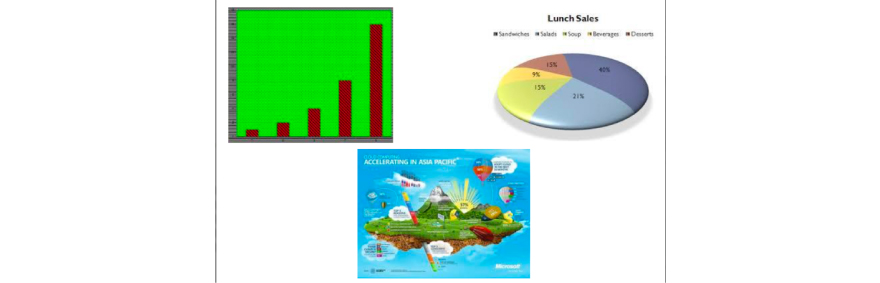Three common types of chartjunk are as follows:

• Unintentional optical art
• The self-promoting graphical duck

Example of unintentional optical art can be shown as per the example below.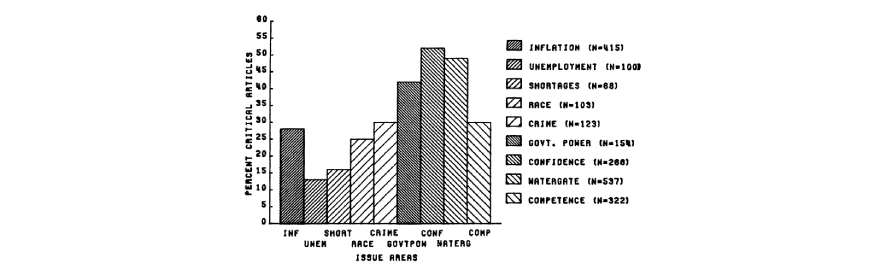These are nothing but illusions and unwanted effects rather than conveying what should be ideally conveyed.

Example of the dreaded grid can be shown as per the example below.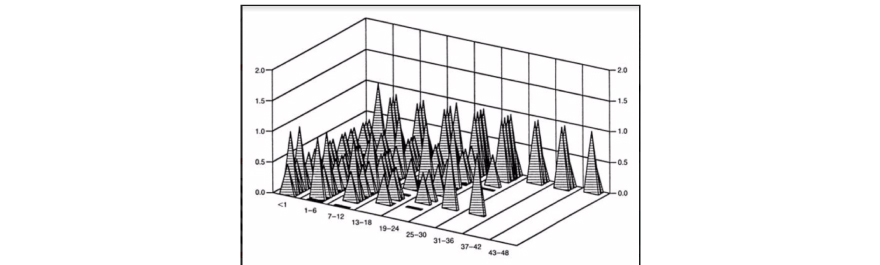If we look at it – gridlines convey no information, dark gridlines are chartjunk. If gridlines are needed, they should be light grey.

Why do we create chartjunk – primarily because of the following aspects:

• Lack of quantitative skills of professional artists
• The belief that statistical data are boring
• Graphics are only for the unsophisticated reader

Response:

Options a, b, c, d is all correct. All of these can be used to remove the legend.

We use legendTest + guides(fill=FALSE) to remove legend for a particular aesthetic. This can also be possible in option b which is using the scale_fill_discrete() function when specifying the scale.

The third option in option c which is legendTest + theme(legend.position="none") will remove all legends in the plot.

Option d also has similar syntax format as in option a which will enable to remove the legend.

Response:

Yes, trend lines can be added into the plot in R.

Below is an example where we have added a vertical line as the mean of the variable for determining the threshold into the histogram plot that we have plotted using the iris dataset in R.

The ggplot2 library in R is leveraged for this purpose.

library(ggplot2)

ggplot(data = iris,aes(x=Sepal.Length))+geom_histogram(fill="lightblue",col="blue")+geom_vline(xintercept = mean(iris$Sepal.Length),color="red",linetype="longdash") The function geom_vline where the line stands for the vertical line is used. Here we just need to provide the intercept on x-axis only. The mean of Sepal.Length parameter is taken as a threshold to determine where the line has to be drawn. The type of the line can also be determined as shown by using the parameter “linetype”.Response: The correct answer is c and a. Both “table” and “xtabs” can be used to accomplish this. “Table” is the one that uses cross-specifying factors to build a contingency table of the counts at each combination of factor levels.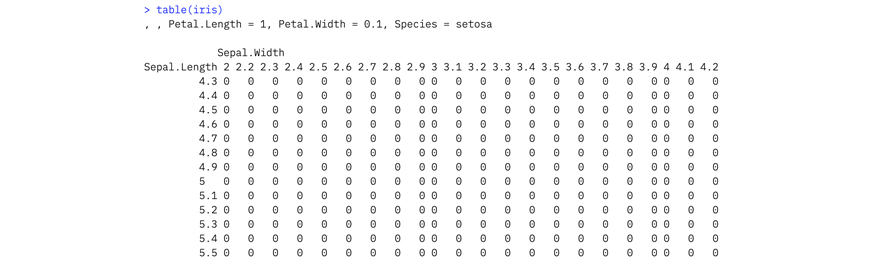Xtabs also creates a contingency table(optionally a sparse matrix) from cross-classifying factors, usually contained in a data frame, using a formula interface. List is used as a function to construct, coerce and check for both kinds of R lists. Stem produces a stem and leaf plot of the values. It is used for a different purpose than what is requested here. It uses parameter such as “scale” that can be used to expand the scale of the plot. Response: Correct answer is Option b – bwplot() Bwplot() is the Box and Whisker plot used for numerical variables. This is part of lattice package in R. Below is an example of a box and whisker plot using the singer dataset.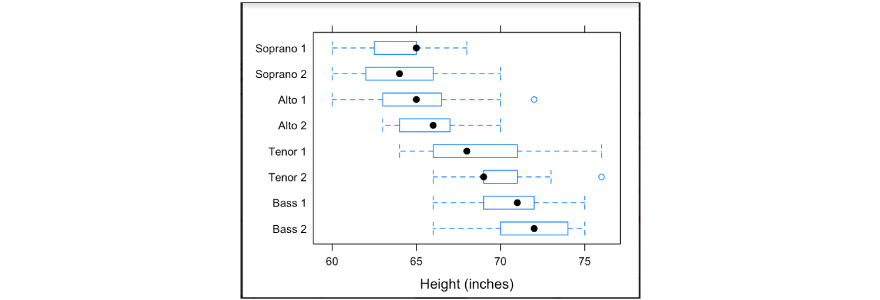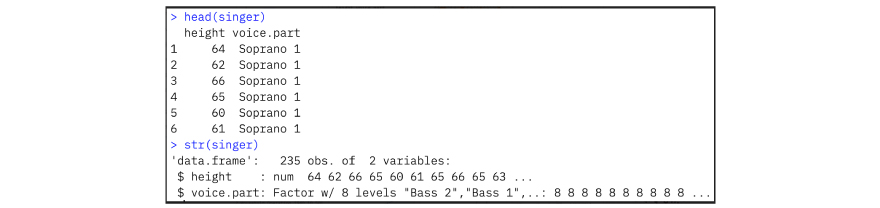library(lattice) require(stats) #bwplot bwplot(voice.part ~ height, data=singer, xlab="Height (inches)") plot() is used for generic x-y plotting. xyplot() produces bivariate scatterplots or time-series plots. #xyplot ## Tonga Trench Earthquakes Depth <- equal.count(quakes$depth, number=8, overlap=.1)
xyplot(lat ~ long | Depth, data = quakes)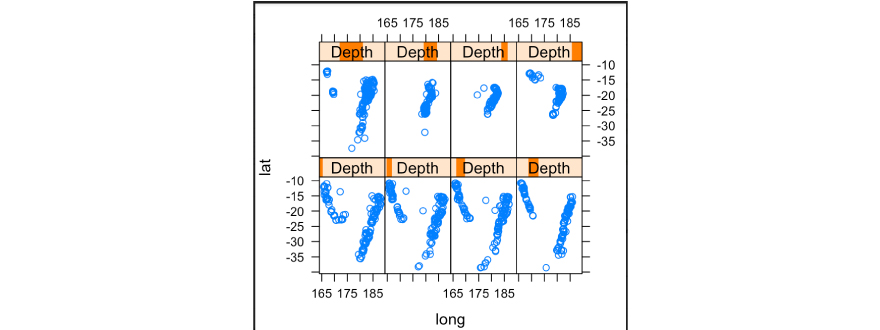dotplot() produces Cleveland dot plots.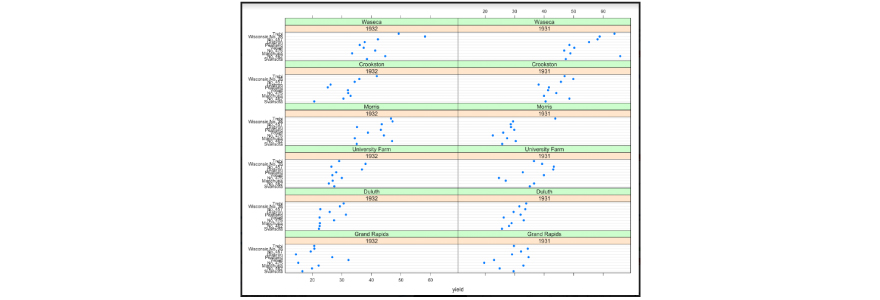Response:

We can use Prop.table() that computes proportions from a contingency table.• Margin.table() is used for a contingency table in array form, compute the sum of table entries for a given index.
• Addmargins() – puts arbitrary margins on multidimensional tables or arrays

For a given table one can specify which of the classifying factors to expand by one or more levels to hold margins to be calculated. One may for example form sums and means over the first dimension and medians over the second. The resulting table will then have two extra levels for the first dimension and one extra level for the second. The default is to sum over all margins in the table. Other possibilities may give results that depend on the order in which the margins are computed. This is flagged in the printed output from the function.

This is a common yet one of the most important data visualization in R interview questions and answers for experienced professionals, don't miss this one.

Response:

We can use a q-q plot for this.

Let us take an example.

• set.seed(123)
• # Normally distributed numbers
• x <- rnorm(100, mean=50, sd=5)
• # Uniformly distributed numbers
• z <- runif(100)

We can compare the numbers sampled with rnorm() against normal distribution.

• qqnorm(x)
• qqline(x)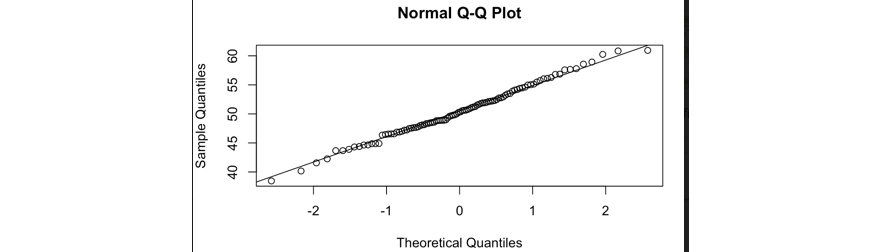We can then experiment with the same numbers to the 3rd power, compared to the normal distribution.

• qqnorm(x^3)
• qqline(x^3)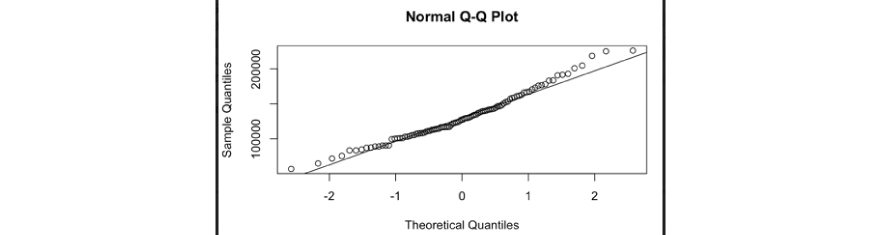Numbers sampled from the flat distribution, compared to normal is described below.

• qqnorm(z)
• qqline(z)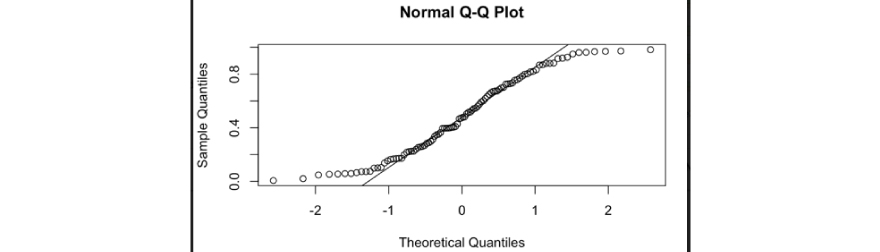Response:

We can publish our visualization as a standalone HTML page using the publish method. Currently, we can publish our chart as a gist or to rpubs.

For example:

• names(iris) = gsub("\\.", "", names(iris))
• r1 <- rPlot(SepalLength ~ SepalWidth | Species, data = iris,
• color = 'Species', type = 'point')
• r1$publish('Scatterplot', host = 'gist') • r1$publish('Scatterplot', host = 'rpubs')
• rCharts can also be embedded into a Shiny application using the utility functions renderChart and showOutput.
• rCharts can also be embedded into an Rmd document using knit2html or in a blog post using slidify.
• rCharts is licensed under the MIT License. The JavaScript charting libraries that are included with this “rCharts” package are licensed under their own terms. All of them are free for non-commercial and commercial use, with the exception of Polychart and Highcharts, both of which require paid licenses for commercial use.

Response:

The package “slidify” helps create and publish HTML5 presentations from RMarkdown. Slidify is designed to be modular and provides a higher degree of customization for the more advanced user.

We can access defaults using slidifyDefaults(). It is possible to override options by passing it to slidify as a named list or as a yaml file.

• Framework: slide generation framework to use
• theme: theme to use for styling slide content
• Highlighter: a tool to use for syntax highlighting
• hitheme: style to use for syntax highlighting
• mode: selfcontained, standalone, draft
• URL: paths to lib
• Widgets: widgets to include

Slidify makes it easy to create, customize and publish, reproducible HTML5 slide decks from R Markdown.  It is designed to make it very easy for an HTML novice to generate a crisp, visually appealing HTML5 slide deck, while at the same time giving advanced users several options to customize their presentation.

Response:

Each and every visualization in ggplot2 package in R comprises of the following key aspects –

• Data – The raw material of your visualization
• Layers – What you can see or visualize on plots (i.e. lines, points, maps etc.)
• Scales – Maps the data to graphical output
• Coordinates – This is from the visualization perspective (i.e. grids, tables etc.)
• Faceting – Provides “visual drill-down” into the data
• Themes – Controls the details of the display (i.e. fonts, size, colour etc.)
1. Response:

To generate facet row-wise, we can do the following:

ggplot(data = mpg) + geom_point(mapping = aes(x = displ, y = hwy)) + facet_grid(drv ~ .)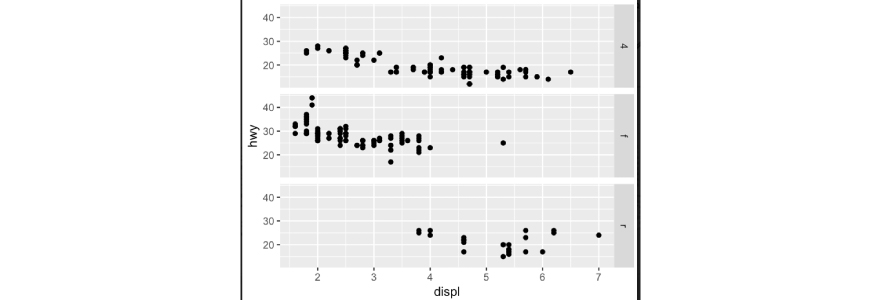To generate facet column-wise, we can do the following:

ggplot(data = mpg) + geom_point(mapping = aes(x = displ, y = hwy)) + facet_grid(.~ drv)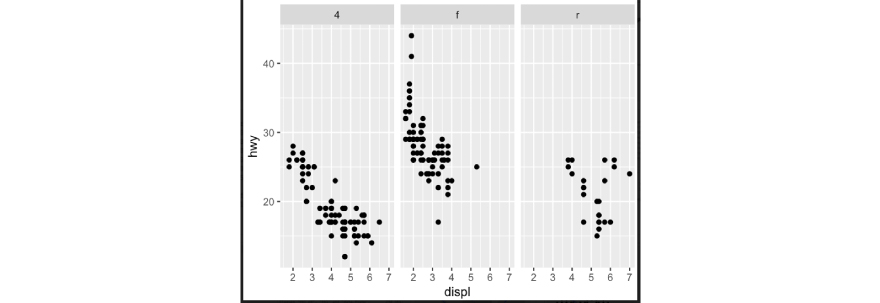Response:

If we look at the dataset, the frequency of distribution has to be plotted as a histogram with the help of the ggplot2 library in R. We can consider “cut” parameter which categorizes required information.

When we use the “table” command, then we can get an idea of a number of records, whether there are missing values (here, in this case, there are no missing values) and henceforth it can be used to plot the histogram chart.We can use the geom_bar function and using “cut” parameter in the x-axis to display the necessary information as per below.

library(ggplot2)
attach(diamonds)
str(diamonds)
ggplot(data = diamonds)+geom_bar(mapping = aes(x = cut))We see that desired plot is represented and we are also able to validate values at a high level based on the “table” command that we had used to get an understanding of the distribution of the data information.

Don't be surprised if this question pops up as one of the top interview questions for data visualization in R in your next interview.

Response:

The above chart represents the “toothgrowth” data analysis between length vs dose, given type of supplement.

The supplement type can be OJ – Orange Juice or VC – Vitamin C. Based on this the plot shows length vs dose comparison for each of the supplement types of categories.

We can accomplish this using coplot() function in R.

• require(graphics)
• coplot(len ~ dose | supp, data = ToothGrowth, panel = panel.smooth,
• xlab = "ToothGrowth data analysis")

Response:

We can create maps using geom_map() function and using expand_limits which takes longitude and latitude parameters of a data frame.

Geom_map is a pure annotation, so does not affect position scales.

We take an example of USArrests dataset in R. This data set contains statistics, in arrests per 100,000 residents for assault, murder, and rape in each of the 50 US states in 1973. Also given is the percent of the population living in urban areas.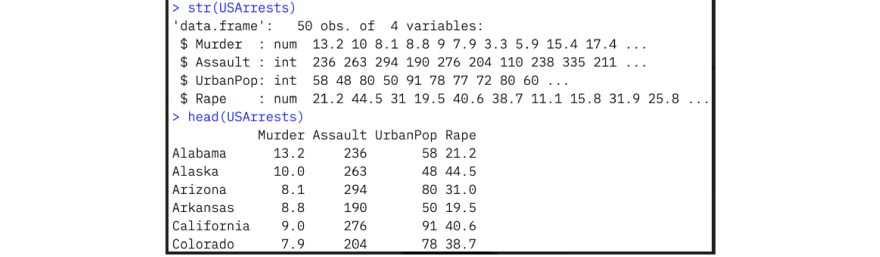We would like to first create a data frame with the information required to be plotted in a map by states of the US.  And then we use the geom_map() function to take map_id as state and expand limits using GPS coordinates to show the state-wise distribution of the data. This is as follows.

• data <- data.frame(murder=USArrests$Murder,state=tolower(rownames(USArrests))) • map <- map_data("state") • k <- ggplot(data,aes(fill=murder)) • k+geom_map(aes(map_id=state),map = map)+expand_limits(x=map$long,y=map$lat)Response: Treemaps can be constructed using the googleVis package. This is an R interface to Google Charts API, allowing users to create interactive charts based on data frames. Charts are displayed locally via the R HTTP help server. A modern browser with an Internet connection is required and for some charts Flash. The data remains local and is not uploaded to Google. Treemaps are usually rectangles placed adjacent to each other. The size of each rectangle is directly proportional to the data being used in the visualization. Treemaps have been used to plot the news on the web by Newsmap.jp. They have also been applied in financial websites such as smart money to visualize financial market movements. Response: Pyramid plots are horizontal bar plots. It displays a pyramid (opposed horizontal bar) plot on the current graphics device. They are typically used in news or journal articles. They are often used to display gender differences. We can achieve plotting this using “plotrix” and “RColorBrewer” packages in R. Below is an example of a pyramid plot for the Australian population for 2002 by gender and by different age groups.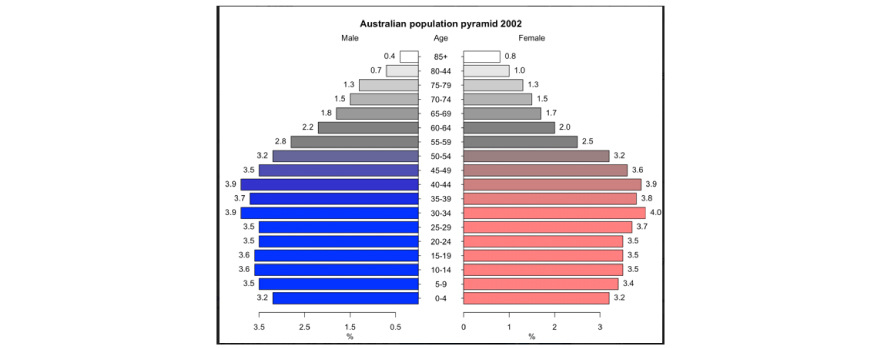## Advanced A linear model can be created on top of an existing scatter plot chart by using geom_smooth() function using ggplot2 library in R. For example: if we consider airquality dataset in R and use ggplot2 to scatter plot between multiple variables such as wind and temperature, then we can notice how linear models can be included in the chart by using geom_smooth().ggplot(data = airquality,aes(y=Wind,x=Temp))+geom_point()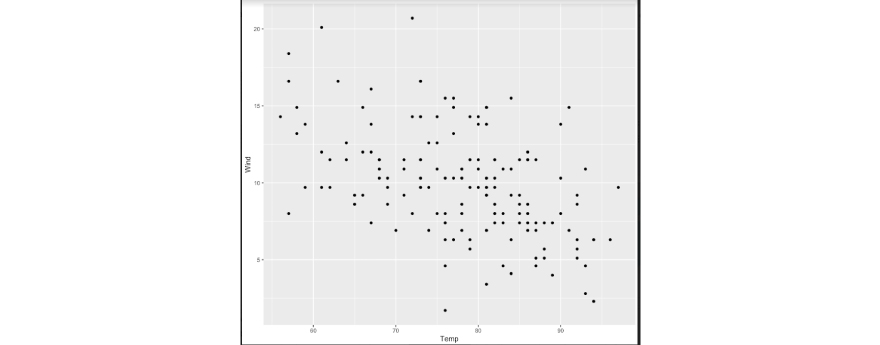ggplot(data = airquality,aes(y=Wind,x=Temp))+geom_point()+geom_smooth(method = "lm")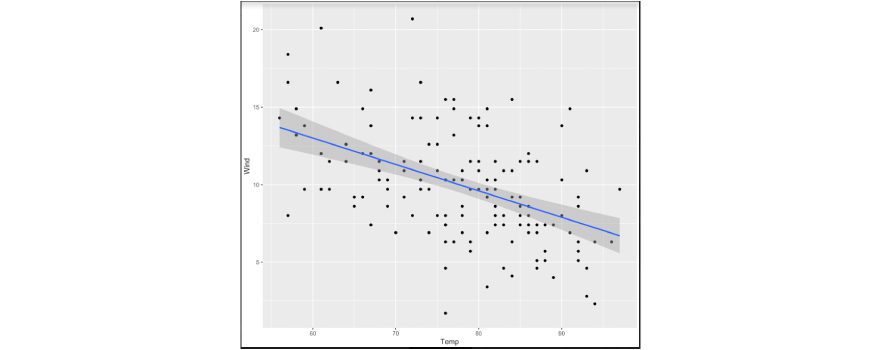Code Snippet 1: library(leaflet) x <- leaflet() %>% addTiles() %>% addMarkers(lng=174.768, lat=-36.852) x Code Snippet 2: library(leaflet) y <- leaflet() %>% addTiles() y The first code snippet will provide a map chart with that of the GPS coordinates as mentioned in addMarkers() function with the parameter of latitude and longitude specifics.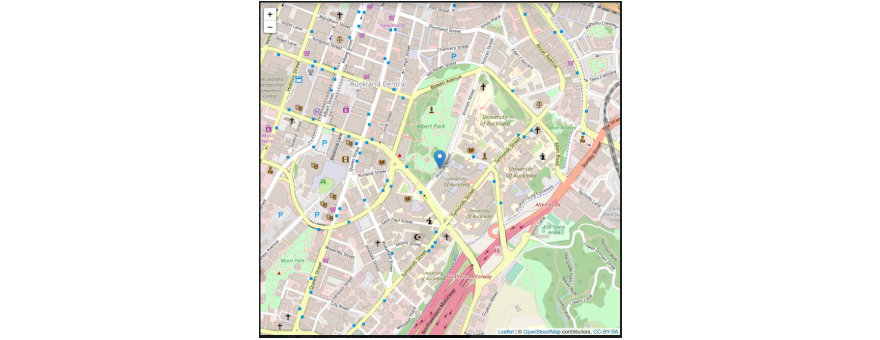The second code snippet will only display a blank map from “OpenStreetMap” based on the features of the leaflet library. It will display a generic world map as specifics of GPS coordinates are unknown.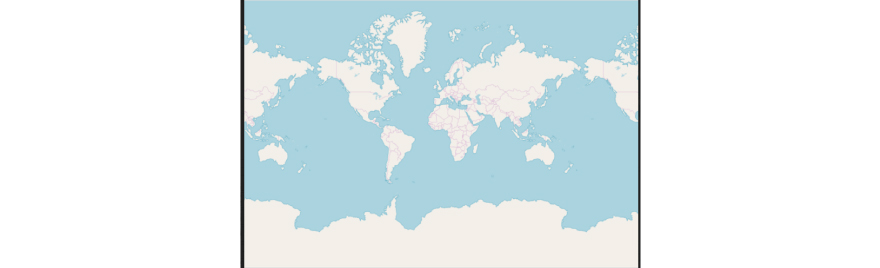This is one of the most frequently asked data visualization in R coding interview questions and answers for freshers in recent times.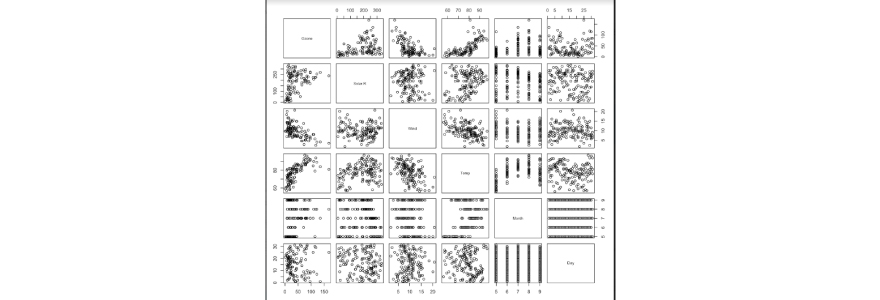When we use plot(airquality) without selecting any particular column or set of columns and when all variables or columns are taken into consideration, then the above chart is displayed. It is a matrix of scatterplots which is nothing but a correlation matrix of all columns in the dataset. Some key inferences are: • If we observe the data representations here, ozone and temperature are correlated positively. • Wind speed is negatively correlated to both ozone and temperature. We can modify charts by tweaking “plot” function by adding the “type” argument. This “type” argument takes the following values: • p – for points • l – for lines • b – for both This will determine the shape of the output graph. For example, if we consider the airquality dataset and plot using these argument options, outputs will be different. # points, lines and both using type argument plot(airquality$Ozone, type= "p") plot(airquality$Ozone, type= "l") plot(airquality$Ozone, type= "b")

Display the only point: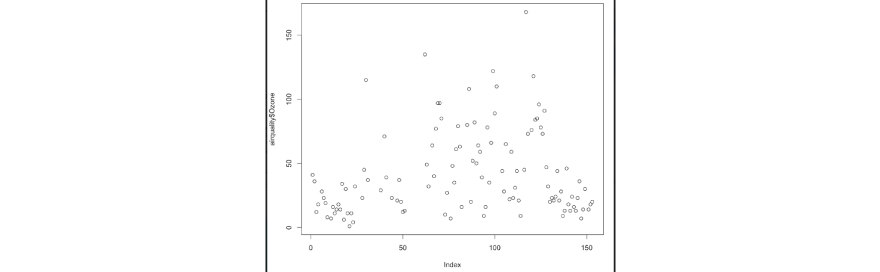Display only line: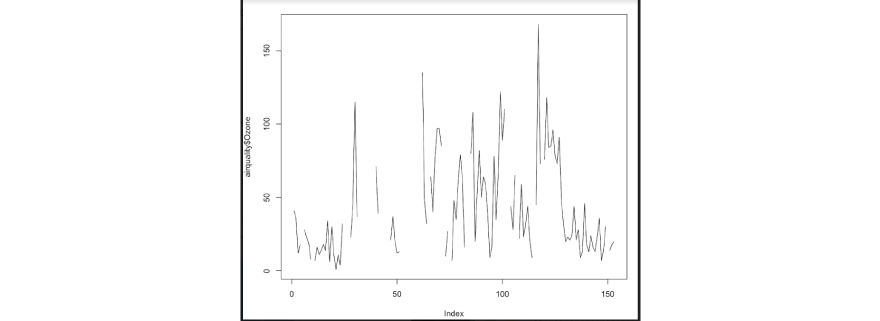Display points and lines (both):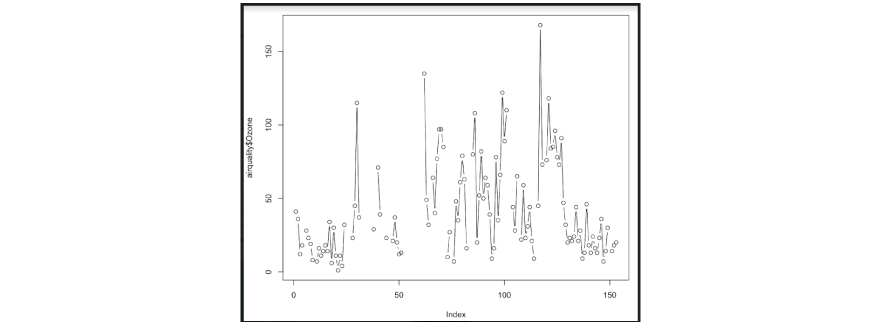Yes, we can create box plots using “plotly” in R.You have to have installed the “plotly” package if it is not installed on your environment and then use the library(plotly) to use it in the session context.

The Orange dataset is used as an example which captures the growth of orange trees information. The box plot is plotted for every tree based on variation in the circumference.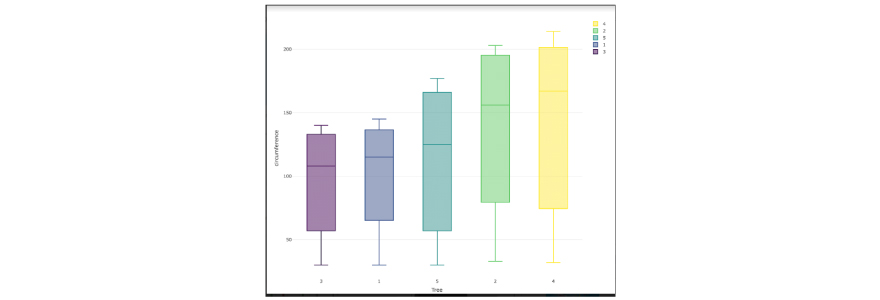Code:

library(plotly) str(Orange) head(Orange)
plot_ly(Orange,y=~circumference,x=~Tree,color=~Tree,type="box")

Expect to come across this, one of the most important data visualization in R interview questions for experienced professionals in data science, in your next interviews.

We can use the RColorBrewer library in R to choose from different colours for different columns in a dataset. We can use “dygraphs” library in R in addition to that. It creates an interactive chart with values can be shown to the point where we hover around our cursor after plotting the graph. The “dygraphs” library in R is having interactive feature out of the box, with default mouse-over labels and we can also perform zooming and panning.

For this, we are considering the “lungDeaths” dataset in R which has deaths from lung disease in the UK captured for a period of few years from 1974 to 1979.

library(dygraphs)
lungDeaths <- cbind(ldeaths, mdeaths, fdeaths)
dygraph(lungDeaths, main = "Deaths from Lung Disease (UK)") %>%
dyOptions(colors = RColorBrewer::brewer.pal(3, "Set2")) Sample chart output: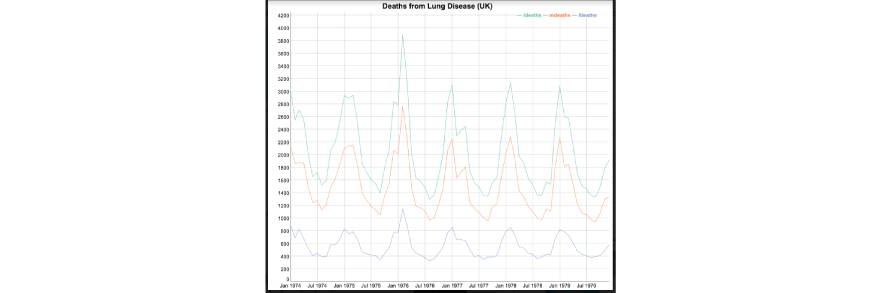Yes, we can create dynamic range selection in the plot in R. For this, we need to leverage the “dygraphs” library and it’s functionality. It offers an interactive range selection capability.

We can use “dyRangeSelector” function to accomplish this.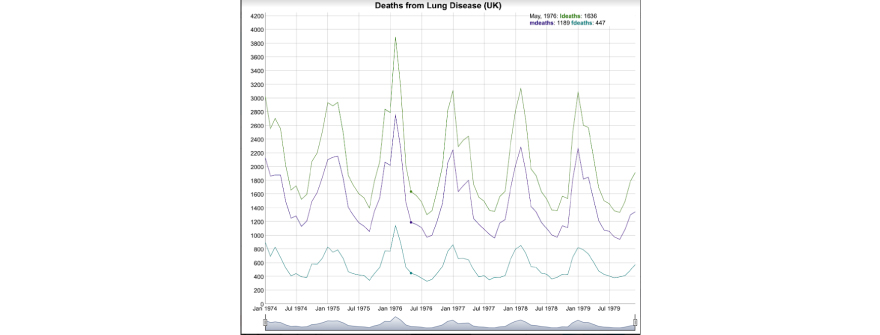dygraph(lungDeaths, main = "Deaths from Lung Disease (UK)") %>%

dyRangeSelector()

We can also use a date range to specify the graph to select that particular range and display accordingly.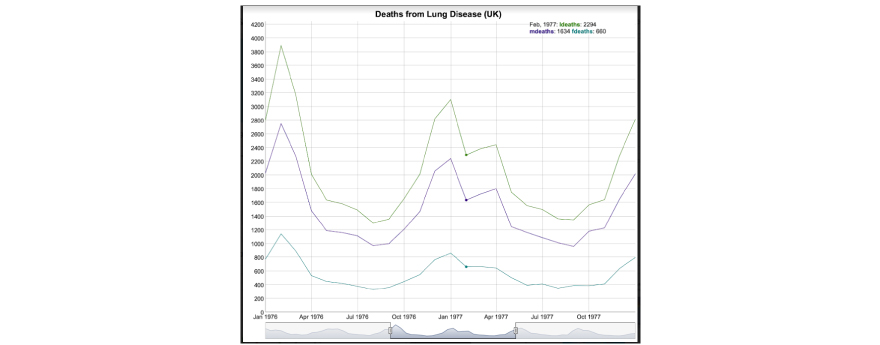These are called “step charts” or “step plots”. The “dygraphs” library in R by default displays time series data in a line.

We can, however, plot the data in a step chart manner by using below function.

library(dygraphs)
lungDeaths <- cbind(mdeaths, fdeaths)
dygraph(lungDeaths, main = "Deaths from Lung Disease (UK)") %>%
dyOptions(stepPlot = TRUE)

We have taken the same “lungDeaths” dataset to display this functionality here.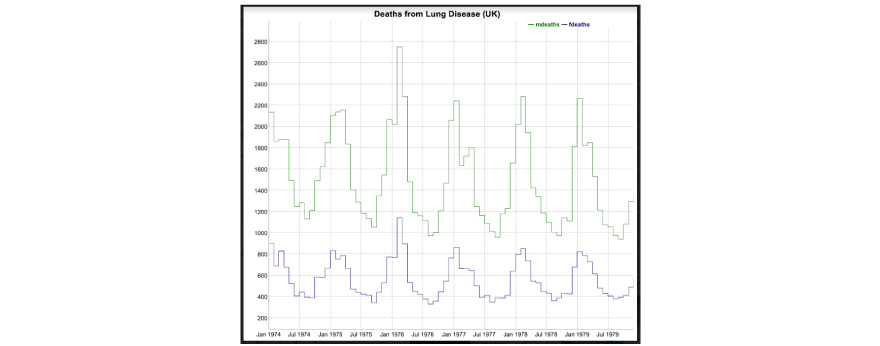Response: We can use “dygraphs” library in R and use functions within it named “dyHighlight” to highlight a particular series where the mouse is hovered on.

We take the lungDeaths sample dataset where there are multiple parameters with time-series data. We can use “dyHighlight” function to accomplish highlighting a particular series when selected as shown below. We can specify here a larger circle size for point highlighting as well as more decisively fade the non-highlighted series.

library(dygraphs) lungDeaths <- cbind(ldeaths, mdeaths, fdeaths) dygraph(lungDeaths, main = "Deaths from Lung Disease (UK)") %>%
dyHighlight(highlightCircleSize = 5,
highlightSeriesBackgroundAlpha = 0.2, hideOnMouseOut = FALSE)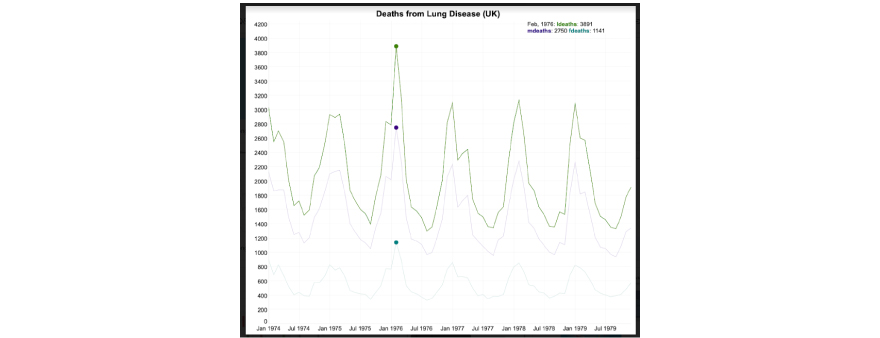Response:

Candlestick charts are interactive charts, primarily used in stock price movements, security or derivative analysis in a real-time or near real-time scenario to describe the price movement.

Yes, it is possible to create such charts in R using libraries such as “plotly” or “dygraphs” and leveraging their function features.

For example, we can take a sample dataset in R from xts which has sample data matrix for simulated 180 observations on 4 variables.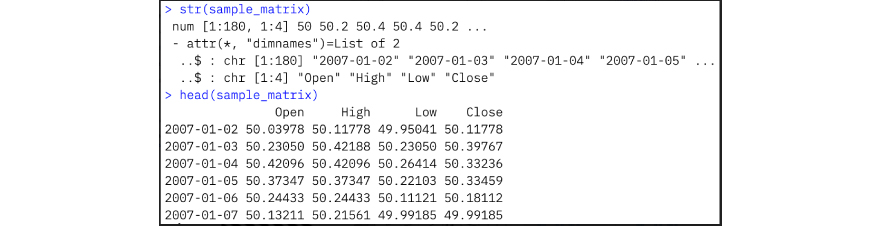Below is a sample output candlestick chart for the above dataset: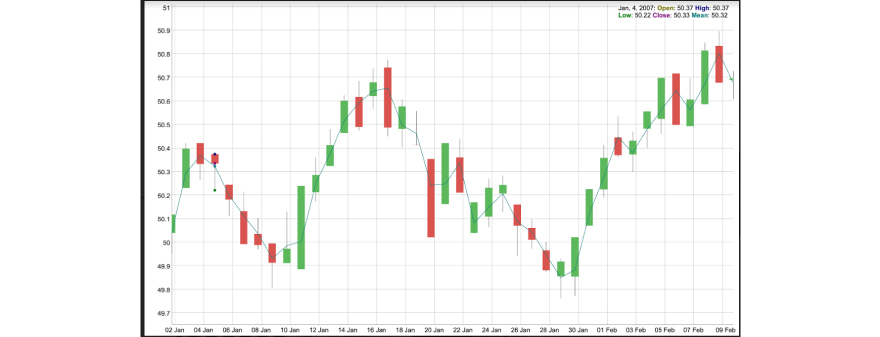Response:

Yes, it will be possible to change the themes/default theme.

By default, ggplot2 creates plots with a greyish background, no axes lines and white grid lines. “ggplot2” was specifically created thinking about scientific publications and user-friendliness. For this reason, its default theme is already perfect for certain scenarios. At the same time, it provides customizable options to change it.

We can add an additional line to change the theme with the function theme_minimal. Here the background is white, we still do not have access lines and the gridlines are coloured in light grey.

We can also choose theme_light. Here we still have a white background and light grey gridlines, however, we also have a grid box around the plot which may be useful in some cases.

We can also have an option as theme_classic. It has a white background no gridlines, and tick black axis lines.

Default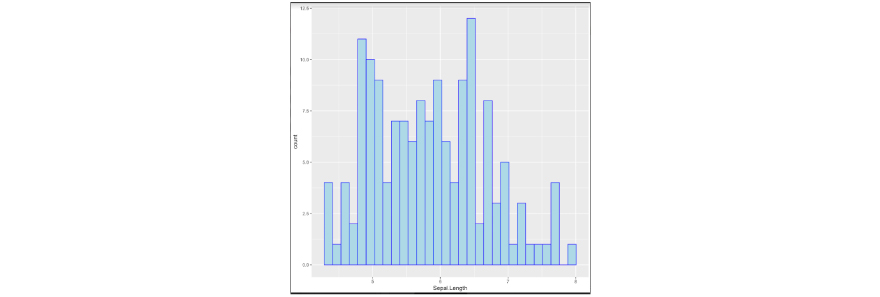With option as theme_minimal():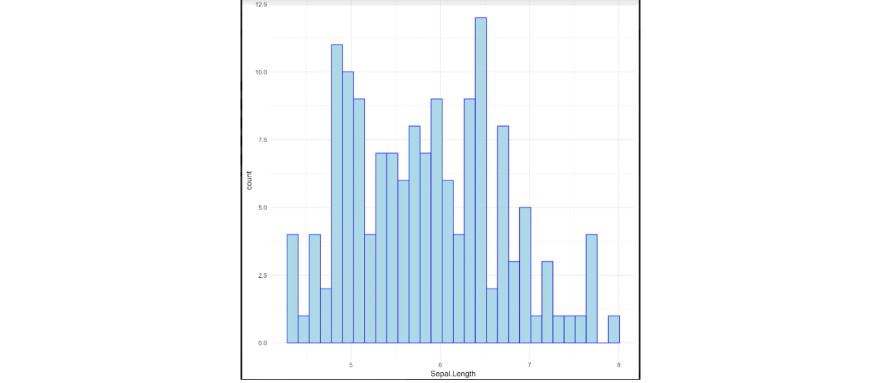With theme_light():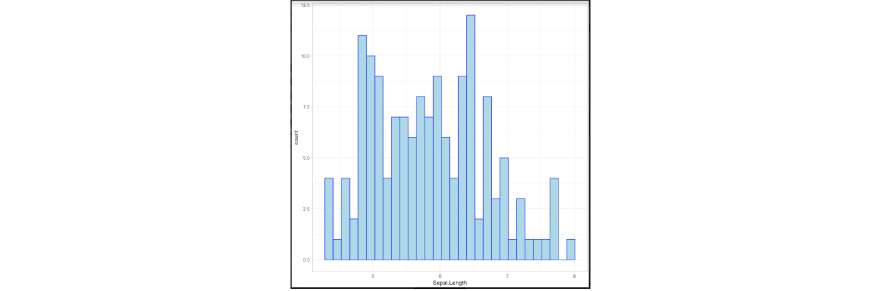With theme_classic():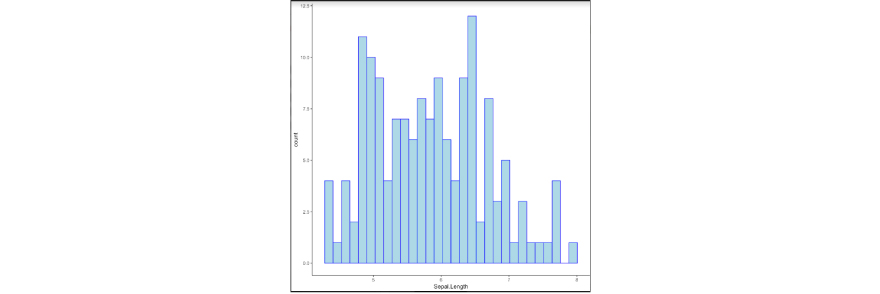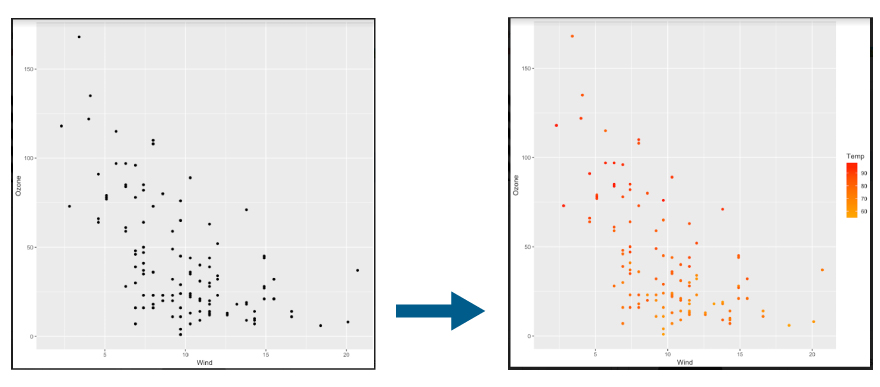Response:

We can accomplish this by using scale_color_gradient() function in the ggplot2 library in R.

• ggplot(data = airquality,aes(x=Wind,y=Ozone))+geom_point()
• ggplot(data = airquality,aes(x=Wind,y=Ozone, color = Temp))+geom_point()+scale_color_gradient(low = "orange",high = "red")

The default colour scale is not always appropriate to spot all the differences in the data we are trying to plot. In many cases, we have to change it so that our plots can become more informative.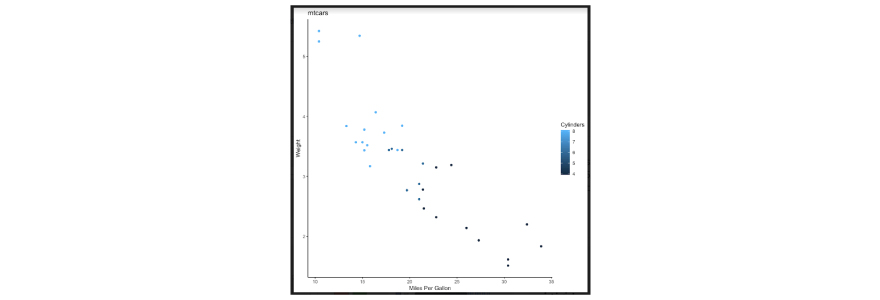Response:

No, the above plot is not the default representation. The axis names and titles are not represented by default and have to be customized with different functions while using ggplot2 libraries in R.

For example, in the above scenario,

• The title “mtcars” won’t appear by default unless it is added by a function called “labs(title = “XXXX….”) in addition to the code for plotting above chart in R
• The X and Y column names will be as per column names defined in the data frame or the dataset which is taken into consideration. i.e. if we consider the “mtcars” dataset, if columns are “wt” and “mpg”, then it will appear that as default. We need to customize to change the label names using – “labs(x=”Miles Per Gallon”, y= “Weight”) etc.
• The colour palette also acts in a similar fashion and it has to be defined.

The above can be accomplished with something as suggested below.

• library(ggplot2)
• p <- ggplot(mtcars, aes(mpg, wt, colour = cyl)) + geom_point()
• p1 <- p + theme_classic() + labs(title = "mtcars", colour = "Cylinders")
• p1 + labs(x = "Miles Per Gallon", y="Weight")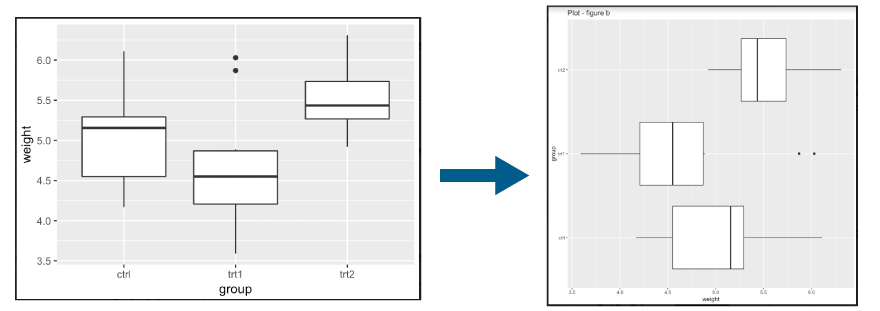a) Using flip() command

b) Using coord_flip() command

c) Using swap() command

d) Using coord_swap() command

e) None of the above, it is not possible in R using ggplot2 libraries

Response:

The correct answer is option b.

You can swap x and y axes using the function coord_flip(). This way x-axis and y-axis can be defined vertical/horizontal and vice versa depending on the columns we choose from the existing dataset.

The below code snippet can be used to represent the plot represented in figure A above. Here weight is shown in y-axis and group information is shown in the x-axis.

• library(ggplot2)
• x <- ggplot(PlantGrowth, aes(x=group, y=weight)) + geom_boxplot()
• x + labs(title = "Plot - figure a")

Now we can use the following to convert the plot to figure b.

• x + coord_flip()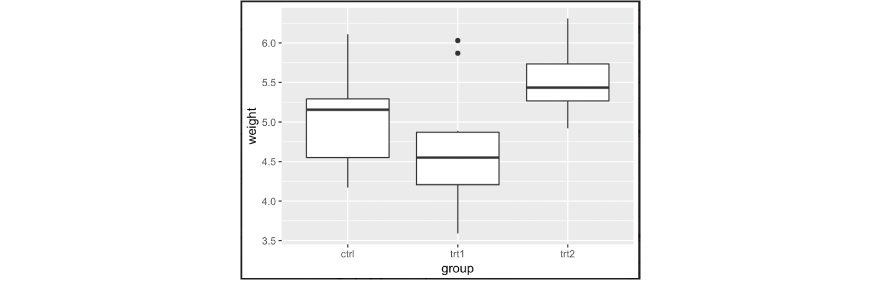Response:

The answer is option A. Yes it is feasible to change the order of items using R.

There are multiple approaches to do it.

Approach 1:

We can manually set the order of a discrete-valued axis. Then we can reverse the order of a discrete value axis and get the levels of the factor. Post this, we can reverse the order and represent the values in a different manner. Example of above is taken and output is shown below.

First consider this.

library(ggplot2)
y <- ggplot(PlantGrowth, aes(x=group, y=weight)) +
geom_boxplot()
y

Then use below code snippet:

# Manually set the order of a discrete-valued axis
y + scale_x_discrete(limits=c("trt1","trt2","ctrl"))
# Reverse the order of a discrete-valued axis
# Get the levels of the factor
flevels <- levels(PlantGrowth$group) flevels # Reverse the order flevels <- rev(flevels) flevels y + scale_x_discrete(limits=flevels) As we can see, the order is changed from (ctrl, trt1, trt2) to (trt2, trt1, ctrl). Approach 2: Alternatively, we can use a built-in function called “scale_x_discrete()” only to accomplish this as per below in a single line command. Sample output is captured below. First consider this. library(ggplot2) y <- ggplot(PlantGrowth, aes(x=group, y=weight)) + geom_boxplot() y Then use below code snippet: y + scale_x_discrete(limits = rev(levels(PlantGrowth$group)))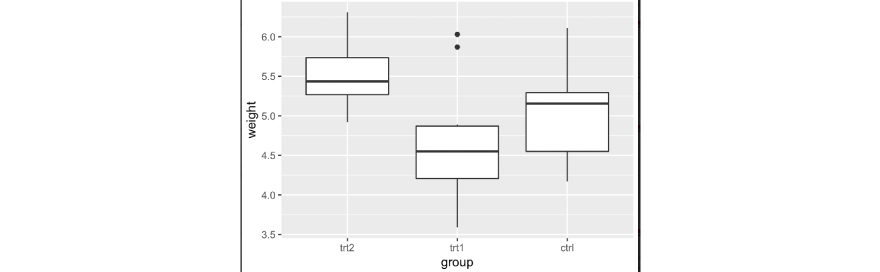As we can see, the order is changed from (ctrl, trt1, trt2) to (trt2, trt1, ctrl).

Response:

We can add supplementary elements such as linear trend lines and quadratic trend lines into the plots in R with the help of the ggplot2 package and its features.

For example, let us consider airquality dataset and we want to draw a scatterplot between two parameters – wind and ozone as per below.

ggplot(data = airquality,aes(x=Wind,y=Ozone))+geom_point()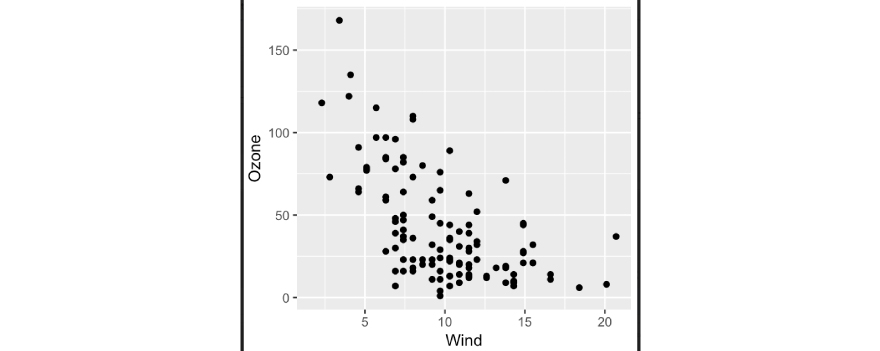We can use the geom_smooth() function and use the “lm” method to draw a linear trend line that is captured based on the current sample data.

ggplot(data = airquality,aes(x=Wind,y=Ozone))+geom_point()+geom_smooth(method = "lm",se=TRUE)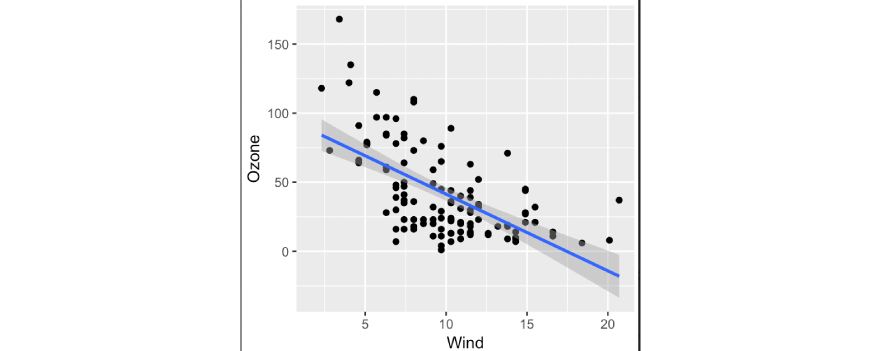Further, we can use a simple quadratic polynomial function to draw a quadratic trend line with the same dataset.

ggplot(data = airquality,aes(x=Wind,y=Ozone))+geom_point()+geom_smooth(method = "lm",formula=y ~ poly(x,2), se=TRUE)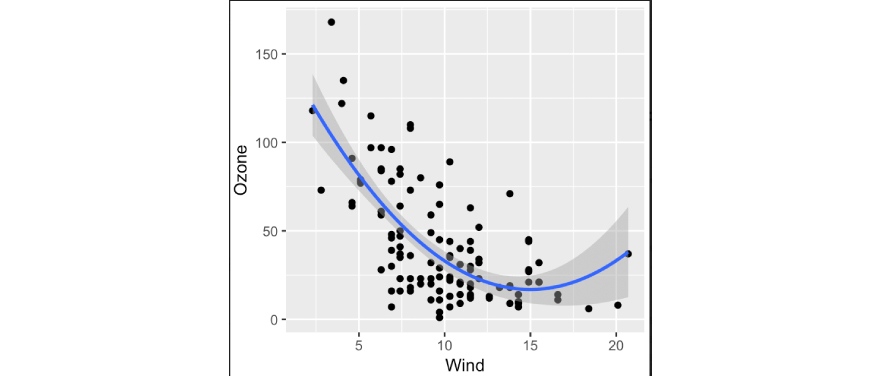Multiplot is regarding showing multiple plots in a chart based on various categorical values. It is possible in R. We can use the function facet_wrap() to accomplish this.

For example, let us consider iris dataset as per below.

ggplot(data = iris,aes(x=Sepal.Length))+geom_histogram(binwidth = 0.1)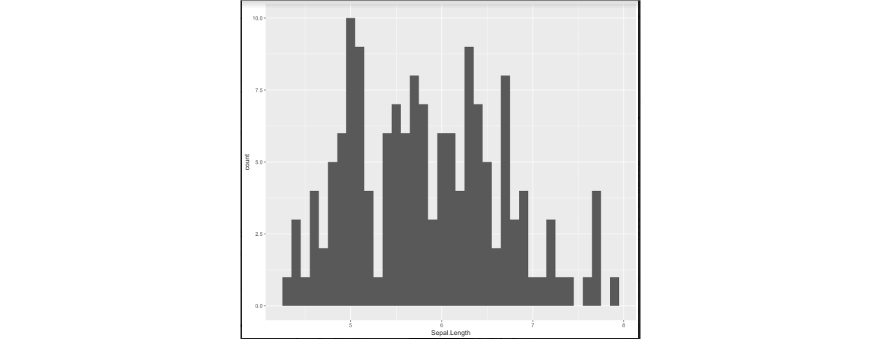ggplot(data = iris,aes(x=Sepal.Length))+geom_histogram(binwidth = 0.1)+facet_wrap(~Species)Above is the example of multi-plot where the histogram is plotted for each categorical values of the parameter – species. The function facet_wrap() function is used for the same.

Response:

We can leverage rCharts to help create interactive visualizations. The design philosophy behind rCharts is to make the process of creating, customizing and sharing interactive visualizations easy.

rCharts uses a formula interface to specify plots, just like the lattice package.

• require(devtools)
• install_github('ramnathv/rCharts')
• require(rCharts)
• names(iris) = gsub("\\.", "", names(iris))
• rPlot(SepalLength ~ SepalWidth | Species, data = iris, color = 'Species', type = 'point')

We can use the iris dataset and use rPlot in rCharts to be able to plot the facetted scatterplots. The output would be something similar to as described below.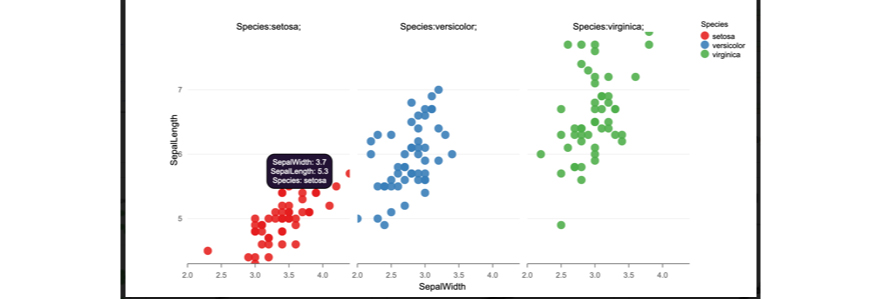Response:

We can leverage rCharts to help create interactive visualizations. The design philosophy behind rCharts is to make the process of creating, customizing and sharing interactive visualizations easy.

rCharts uses a formula interface to specify plots, just like the lattice package.

• require(devtools)
• install_github('ramnathv/rCharts')
• require(rCharts)
• hair_eye = as.data.frame(HairEyeColor)
• rPlot(Freq ~ Hair | Eye, color = 'Eye', data = hair_eye, type = 'bar')

We can use the haireyecolor dataset and use rPlot in rCharts to be able to plot the facetted bar plots in R. The output is described below.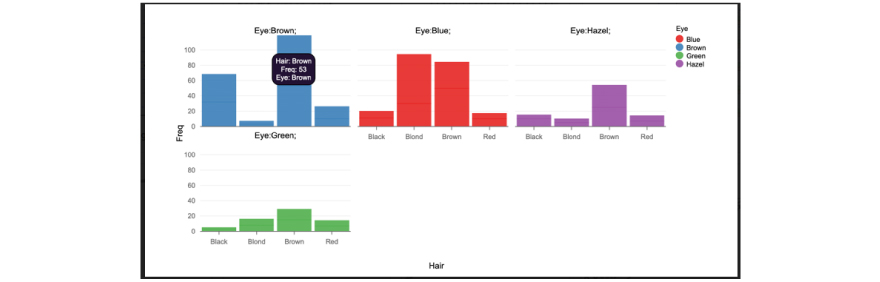Response:

This can be accomplished using the reshape2 package which uses efficient reshaping of data leveraging “data.tables”.

require(reshape2)
uspexp <- melt(USPersonalExpenditure)
names(uspexp)[1:2] = c('category', 'year')
solution <- xPlot(value ~ year, group = 'category', data = uspexp,
type = 'line-dotted')`

solution

We can use XCharts by picking up category and year parameters and use the xPlot() to accomplish the desired plot representation as shown in below output chart.Response:

This is an example of interactive charts that will be created which will represent two series. Each of the series will be plotted based on interactive javascript feature visualizations with the help of rCharts package in R.

Two series will contain data and plot points as specified in the data that it takes as input in the code above.

• For series 1, data = c(1, 3, 2, 4, 5, 4, 6, 2, 3, 5, NA)
• For series 2, data = c(NA, 4, 1, 3, 4, 2, 9, 1, 2, 3, 4)

NA will not have any values and hence plot will not be drawn for that point. Each series has 10 data points and 1 point value as NA. Hence each will display value for 10 data points. Dash style of the plot for each series will be different.

The legends will also be displayed.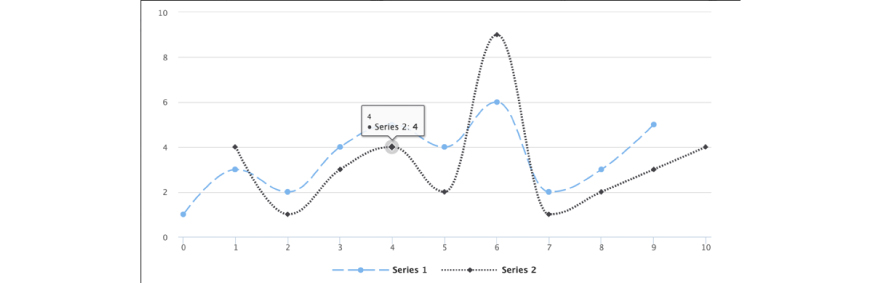rCharts is licensed under the MIT License. The JavaScript charting libraries that are included with this “rCharts” package are licensed under their own terms. All of them are free for non-commercial and commercial use, with the exception of Polychart and Highcharts, both of which require paid licenses for commercial use.

Response:

Equation 1 – will generate a smooth line curve as per below.

Here, line types are segregated by 3 categories of a drive (front-wheel drive, rear-wheel drive, 4 wheel drive) and they are represented by these 3 separate lines. The legend will also appear by default.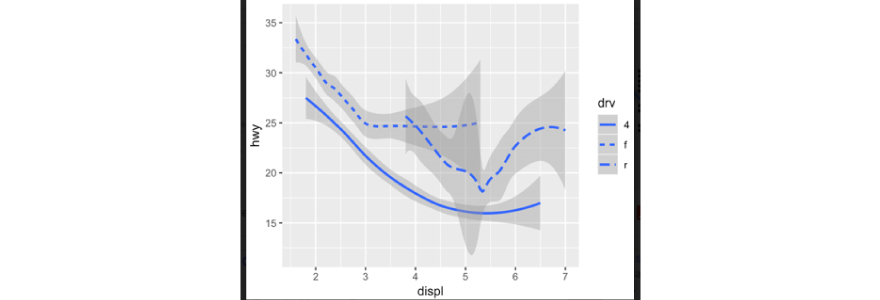Equation 2 – this is used to display multiple geoms. Here the consideration is represented based on values between parameters – hwy and displ.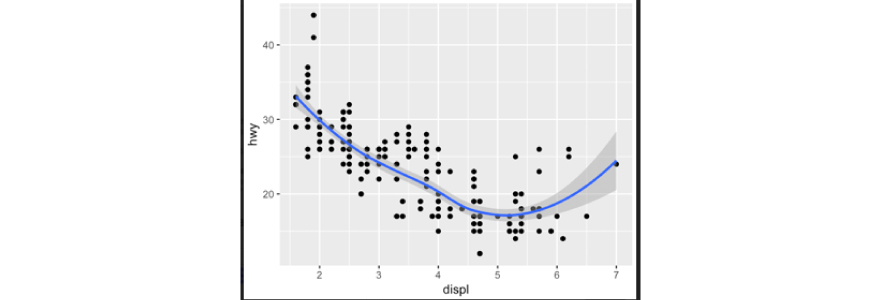Equation 3 – This will generate a colour line curve graph and the colour will be driven by the parameter “drv”. Legends are also going to be displayed mandatorily as there is a parameter which has indicated the same.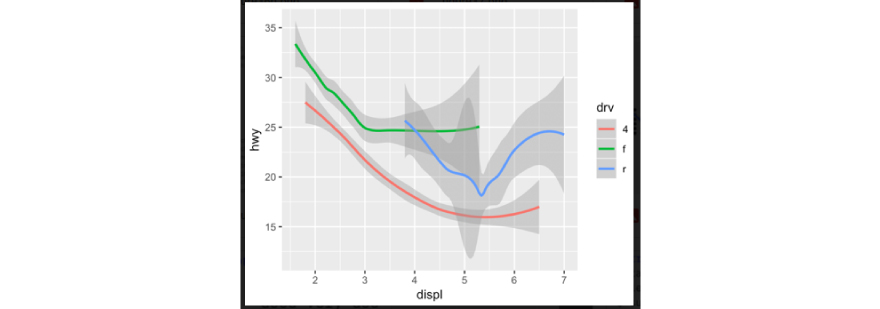Response:

Scenario a:

We have two variables – both continuous. Let’s say continuous variable a and continuous variable b.

We can consider mpg dataset and leverage various functions to analyze data distribution.

i) geom_label() - geom_label() draws a rectangle behind the text, making it easier to read. Example of a plot is shown below.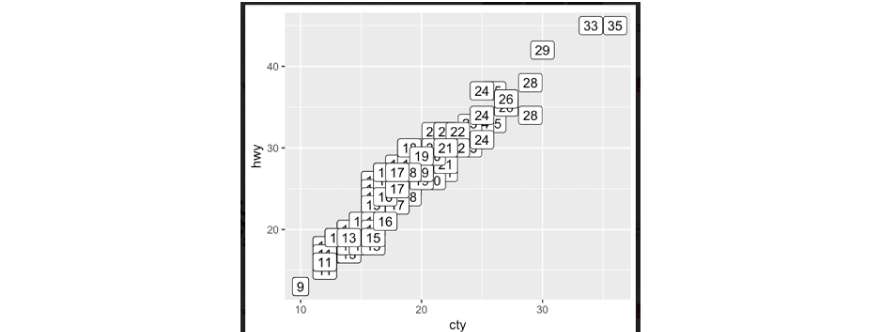a <- ggplot(mpg, aes(cty,hwy))

a+geom_label(aes(label=cty),nudge_x = 1,nudge_y = 1)

i) geom_jitter() - The jitter geom is a convenient shortcut for geom_point(position = "jitter"). It adds a small amount of random variation to the location of each point, and is a useful way of handling overplotting caused in smaller datasets.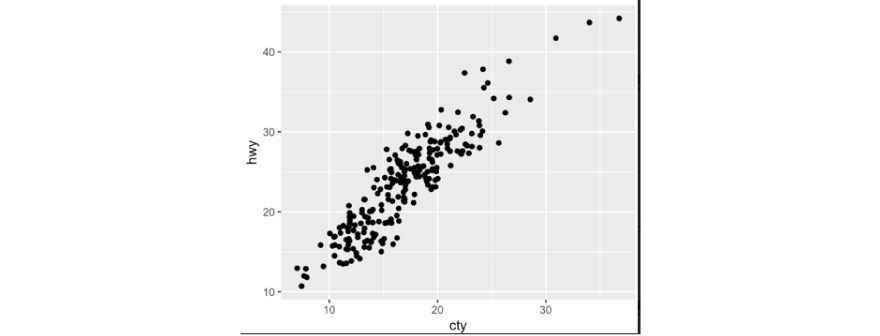a <- ggplot(mpg, aes(cty,hwy))

a+geom_jitter(height = 2,width = 2)

Other usages could be – geom_quantile(), geom_smooth() etc.

Scenario b:

We have two variables – one discrete and other continuous.

We can consider mpg dataset and leverage various functions to analyze data distribution.

i) Geom_col() - There are two types of bar charts: geom_bar() and geom_col(). If you want the heights of the bars to represent values in the data, use geom_col(). geom_col() uses stat_identity(): it leaves the data as-is.

Here when we take “class” and “hwy” parameters in the mpg dataset, we can plot something like below.b <- ggplot(mpg,aes(class,hwy))

b+geom_col()

i) Geom_boxplot() - The boxplot compactly displays the distribution of a variable. It visualises five summary statistics (the median, two hinges and two whiskers), and all "outlying" points individually.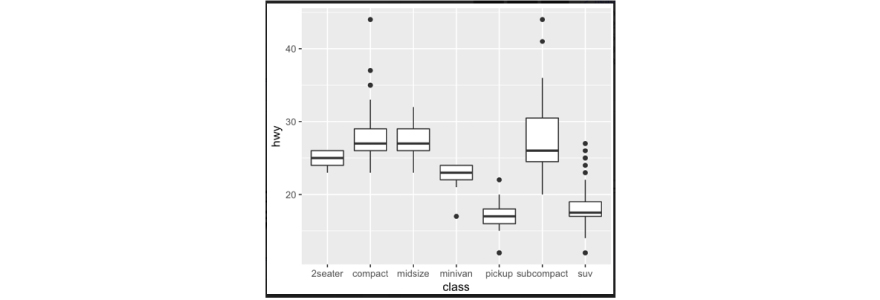b <- ggplot(mpg,aes(class,hwy))

b+geom_boxplot()

Other usages could be – geom_dotplot(), geom_violin() etc.

Response:

We can leverage ggplot2 package for this. Following functions can be used:

• Geom_bin2d() – It divides the plane into rectangles, counts the number of cases in each rectangle, and then (by default) maps the number of cases to the rectangle's fill. This is a useful alternative to geom_point() in the presence of overplotting.
• Geom_density2d() – It performs a two dimensional(2d) kernel density estimation using MASS::kde2d() and display the results with contours. This can be useful for dealing with overplotting. This is a 2d version of geom_density().
• Geom_hex() – It divides the plane into regular hexagons, counts the number of cases in each hexagon, and then (by default) maps the number of cases to the hexagon fill. Hexagon bins avoid the visual artefacts sometimes generated by the very regular alignment of geom_bin2d().

If we take the example of the diamond dataset, below are sample output charts for each type of functions.

Using geom_bin2d():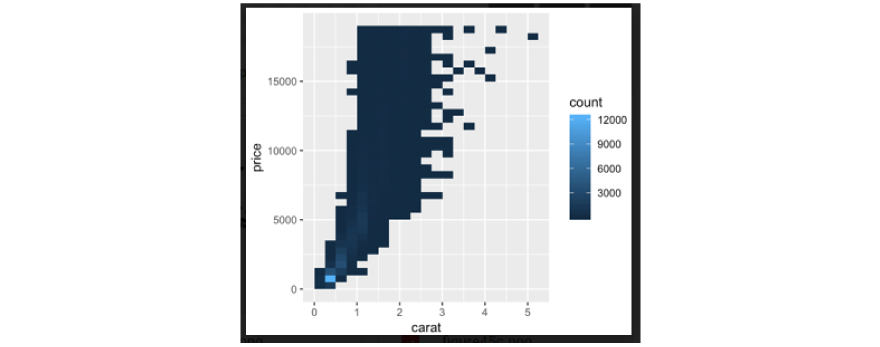Using geom_density2d():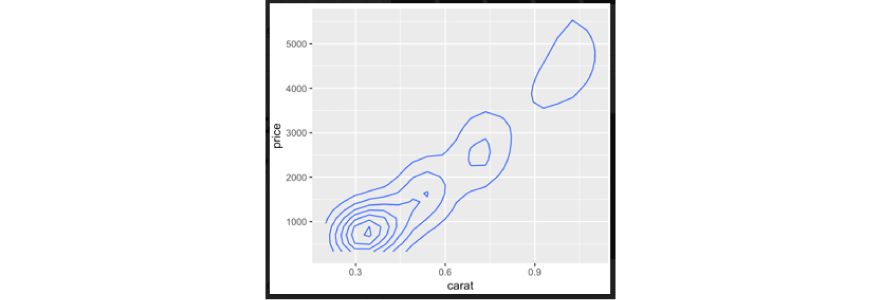Using geom_hex():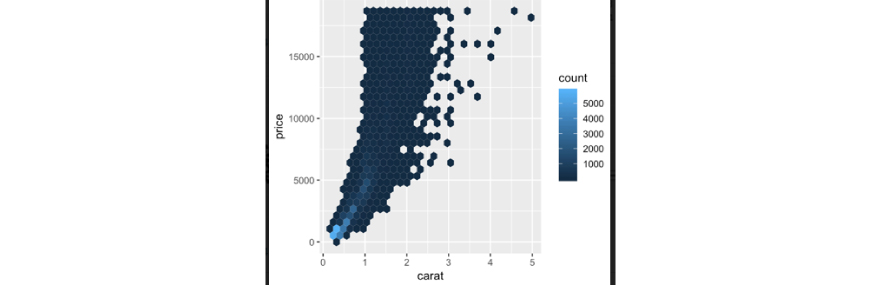Response:

All of the options are correct. Select all 4 options.

Geom_crossbar() - Various ways of representing a vertical interval defined by x, ymin and ymax. Each case draws a single graphical object. We can try below example to explain this.

df <- data.frame(grp=c("A","B"),fit=4:5,se=1:2)

j <- ggplot(df,aes(grp,fit,ymin=fit-se,ymax=fit+se))

j+geom_crossbar(fatten = 2)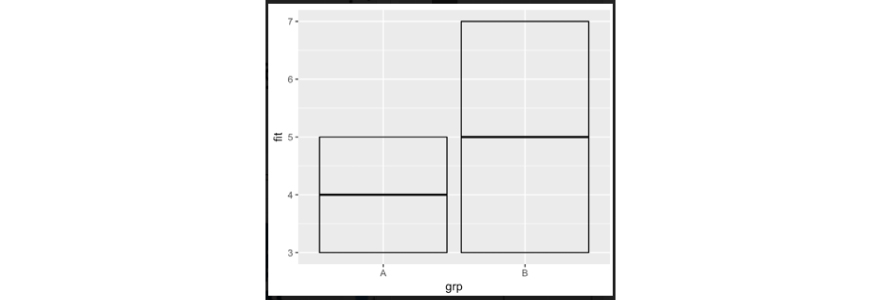Geom_errorbar() – It is a rotated version of geom_crossbar() and we can observe that the error details can be visualized.

df <- data.frame(grp=c("A","B"),fit=4:5,se=1:2)

j <- ggplot(df,aes(grp,fit,ymin=fit-se,ymax=fit+se))

j+geom_errorbar()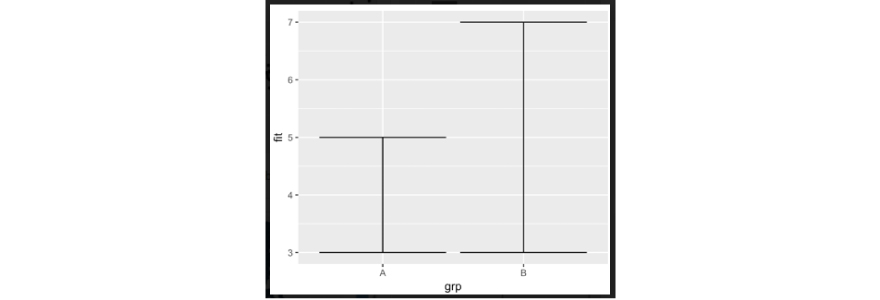Geom_linerange() – It is an extended feature of geom_crossbar() and we can observe that the error details can be visualized in a different manner.

df <- data.frame(grp=c("A","B"),fit=4:5,se=1:2)

j <- ggplot(df,aes(grp,fit,ymin=fit-se,ymax=fit+se))

j+geom_linerange()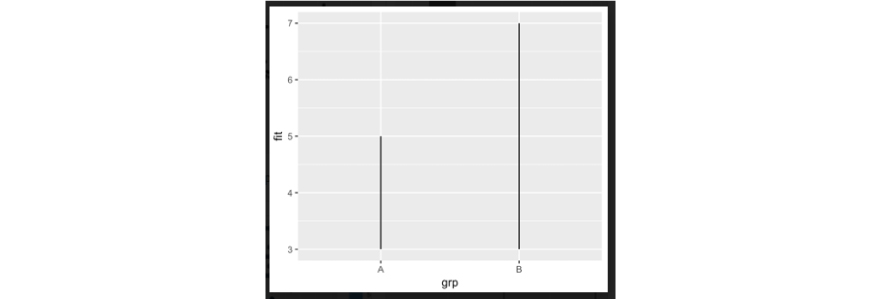Geom_pointrange() – It is an extended feature of geom_crossbar() and we can observe that the error details can be visualized in a different manner.

df <- data.frame(grp=c("A","B"),fit=4:5,se=1:2)

j <- ggplot(df,aes(grp,fit,ymin=fit-se,ymax=fit+se))

j+geom_pointrange()